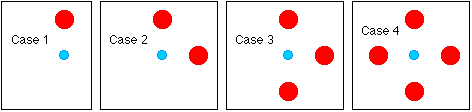#### Question

A small blue ball has 1 or more large red balls placed near it. The red balls are all the same mass and the same distance from the blue one. Rank the different cases based on the net gravitational force experienced by the blue ball.The correct ranking, from largest net force to smallest, is:

1. 1=3>2>4
2. 3>2>1>4
3. 4>3>2>1
4. 2>1=3>4
5. 4>1=2=3

#### The Principle of Superposition

What if several objects exert gravitational forces on one another?

The Principle of Superposition: The net force on an object is the vector sum of the individual forces on the object.

In Case 4 shown in the picture, for instance, the net force on the small blue mass in the center can be found by determining the force due to each of the four red masses separately, and then adding the resulting vectors. The red masses exert forces on one another, but those do not matter - all we need to worry about is the force that each red mass applies to the blue one.

#### Gravity Inside the Earth

If we bring a mass from far away toward the Earth, the gravitational force increases...the closer it gets, the bigger the force. This is certainly true when the mass is outside the Earth - what happens if we bring it right to the surface and then keep going, tunneling into the Earth?

If you're inside the Earth, as you get closer to the center of the Earth does the force of gravity increase, decrease, or stay the same?

1. increase
2. decrease
3. stay the same

A parallel question is this one - what is the force of gravity on an object at the center of the Earth?

1. zero
2. infinite

The net force is zero - there's as much mass pulling you one direction as there is pulling the opposite way. The force of gravity decreases as you move from the surface to the center.

This is a consequence of Gauss' Law for Gravity. One implication of this is that inside a spherical shell of mass, the force of gravity due to the shell is zero. Outside the shell, the force is exactly the same as that from a point mass of the same mass as the shell, placed at the center of the shell.